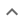•# Factoring multivariable polynomials

We think you wrote:

###This solution deals with factoring multivariable polynomials.

# No solutions found### Try this:

We are constantly updating the types of the problems Tiger can solve, so the solutions you are looking for could be coming soon!

## Step by Step Solution## Step  1  :

#### Equation at the end of step  1  :

  ((a2) +  5ab) +  (2•3b2)  = 0


## Step  2  :

#### Trying to factor a multi variable polynomial :

Factoring    a2 + 5ab + 6b2

Try to factor this multi-variable trinomial using trial and error

Found a factorization  :  (a + 3b)•(a + 2b)

#### Equation at the end of step  2  :

  (a + 3b) • (a + 2b)  = 0


## Step  3  :

#### Theory - Roots of a product :

A product of several terms equals zero.

When a product of two or more terms equals zero, then at least one of the terms must be zero.

We shall now solve each term = 0 separately

In other words, we are going to solve as many equations as there are terms in the product

Any solution of term = 0 solves product = 0 as well.

#### Equation of a Straight Line

Solve   a+3b  = 0

Tiger recognizes that we have here an equation of a straight line. Such an equation is usually written y=mx+b ("y=mx+c" in the UK).

"y=mx+b" is the formula of a straight line drawn on Cartesian coordinate system in which "y" is the vertical axis and "x" the horizontal axis.

In this formula :

y tells us how far up the line goes
x tells us how far along
m is the Slope or Gradient i.e. how steep the line is
b is the Y-intercept i.e. where the line crosses the Y axis

The X and Y intercepts and the Slope are called the line properties. We shall now graph the line  a+3b  = 0 and calculate its properties

#### Graph of a Straight Line :


var c=document.getElementById("myCanvas");var ctx=c.getContext("2d");ctx.beginPath();ctx.moveTo(50,128);ctx.lineTo(280,128);ctx.lineWidth=1;ctx.font="12px Arial";ctx.strokeText("0.000",5,131);ctx.strokeText("b intercept",53,131);ctx.strokeText("0.000",5,131);ctx.strokeText("a = 0.000",180,15);ctx.strokeText("a intercept",173,180);ctx.strokeText("a = 0.000",178,195);ctx.strokeStyle="#e0e0e0";ctx.stroke();ctx.beginPath();ctx.moveTo(203,25);ctx.lineTo(203,128);ctx.moveTo(110,128);ctx.lineTo(203,128);ctx.moveTo(203,128);ctx.lineTo(203,170);ctx.strokeStyle="#e0e0e0";ctx.stroke();ctx.moveTo(50,185);ctx.lineTo(50,15);ctx.lineWidth=1;ctx.strokeStyle="#e0e0e0";ctx.stroke();ctx.beginPath();ctx.strokeStyle="#ff3333";ctx.strokeText("b",25,14);ctx.stroke();ctx.beginPath();ctx.strokeStyle="#ff3333";ctx.strokeText("a",278,130);ctx.stroke();ctx.beginPath();ctx.moveTo(280,185);ctx.lineTo(50,15);ctx.lineWidth=2;ctx.strokeStyle="#7f00ff";ctx.stroke();ctx.beginPath();ctx.lineWidth=1;ctx.moveTo(126,128);ctx.lineTo(126,71);ctx.font="12px Arial";ctx.strokeStyle="#009900";ctx.strokeText(" Rise ",91,99);ctx.stroke();ctx.beginPath();ctx.lineWidth=1;ctx.moveTo(203,128);ctx.lineTo(126,128);ctx.font="12px Arial";ctx.strokeStyle="#ff3333";ctx.strokeText(" Run ",147,143);ctx.stroke();

#### Calculate the Y-Intercept :

Notice that when a = 0 the value of b is 0/3 so this line "cuts" the b axis at b= 0.00000

  b-intercept = 0/3  =  0.00000

#### Calculate the X-Intercept :

When b = 0 the value of a is 0/1 Our line therefore "cuts" the a axis at a= 0.00000

  a-intercept = 0/1  =  0.00000

#### Calculate the Slope :

Slope is defined as the change in b divided by the change in a. We note that for a=0, the value of b is -0.000 and for a=2.000, the value of b is -0.667. So, for a change of 2.000 in a (The change in a is sometimes referred to as "RUN") we get a change of -0.667 - -0.000 = -0.667 in b. (The change in b is sometimes referred to as "RISE" and the Slope is m = RISE / RUN)

    Slope     = -0.667/2.000 = -0.333

#### Equation of a Straight Line

Solve   a+2b  = 0

Tiger recognizes that we have here an equation of a straight line. Such an equation is usually written y=mx+b ("y=mx+c" in the UK).

"y=mx+b" is the formula of a straight line drawn on Cartesian coordinate system in which "y" is the vertical axis and "x" the horizontal axis.

In this formula :

y tells us how far up the line goes
x tells us how far along
m is the Slope or Gradient i.e. how steep the line is
b is the Y-intercept i.e. where the line crosses the Y axis

The X and Y intercepts and the Slope are called the line properties. We shall now graph the line  a+2b  = 0 and calculate its properties

#### Graph of a Straight Line :


var c=document.getElementById("myCbnvas");var ctx=c.getContext("2d");ctx.beginPath();ctx.moveTo(50,128);ctx.lineTo(280,128);ctx.lineWidth=1;ctx.font="12px Arial";ctx.strokeText("0.000",5,131);ctx.strokeText("b intercept",53,131);ctx.strokeText("0.000",5,131);ctx.strokeText("a = 0.000",180,15);ctx.strokeText("a intercept",173,180);ctx.strokeText("a = 0.000",178,195);ctx.strokeStyle="#e0e0e0";ctx.stroke();ctx.beginPath();ctx.moveTo(203,25);ctx.lineTo(203,128);ctx.moveTo(110,128);ctx.lineTo(203,128);ctx.moveTo(203,128);ctx.lineTo(203,170);ctx.strokeStyle="#e0e0e0";ctx.stroke();ctx.moveTo(50,185);ctx.lineTo(50,15);ctx.lineWidth=1;ctx.strokeStyle="#e0e0e0";ctx.stroke();ctx.beginPath();ctx.strokeStyle="#ff3333";ctx.strokeText("b",25,14);ctx.stroke();ctx.beginPath();ctx.strokeStyle="#ff3333";ctx.strokeText("a",278,130);ctx.stroke();ctx.beginPath();ctx.moveTo(280,185);ctx.lineTo(50,15);ctx.lineWidth=2;ctx.strokeStyle="#7f00ff";ctx.stroke();ctx.beginPath();ctx.lineWidth=1;ctx.moveTo(126,128);ctx.lineTo(126,71);ctx.font="12px Arial";ctx.strokeStyle="#009900";ctx.strokeText(" Rise ",91,99);ctx.stroke();ctx.beginPath();ctx.lineWidth=1;ctx.moveTo(203,128);ctx.lineTo(126,128);ctx.font="12px Arial";ctx.strokeStyle="#ff3333";ctx.strokeText(" Run ",147,143);ctx.stroke();

#### Calculate the Y-Intercept :

Notice that when a = 0 the value of b is 0/2 so this line "cuts" the b axis at b= 0.00000

  b-intercept = 0/2  =  0.00000

#### Calculate the X-Intercept :

When b = 0 the value of a is 0/1 Our line therefore "cuts" the a axis at a= 0.00000

  a-intercept = 0/1  =  0.00000

#### Calculate the Slope :

Slope is defined as the change in b divided by the change in a. We note that for a=0, the value of b is -0.000 and for a=2.000, the value of b is -1.000. So, for a change of 2.000 in a (The change in a is sometimes referred to as "RUN") we get a change of -1.000 - -0.000 = -1.000 in b. (The change in b is sometimes referred to as "RISE" and the Slope is m = RISE / RUN)

    Slope     = -1.000/2.000 = -0.500

## Geometric figure: Two Straight Lines

1.   Slope = -1.000/2.000 = -0.500
2.   a-intercept = 0/1 = 0.00000
3.   b-intercept = 0/2 = 0.00000
4.   Slope = -0.667/2.000 = -0.333
5.   a-intercept = 0/1 = 0.00000
6.   b-intercept = 0/3 = 0.00000